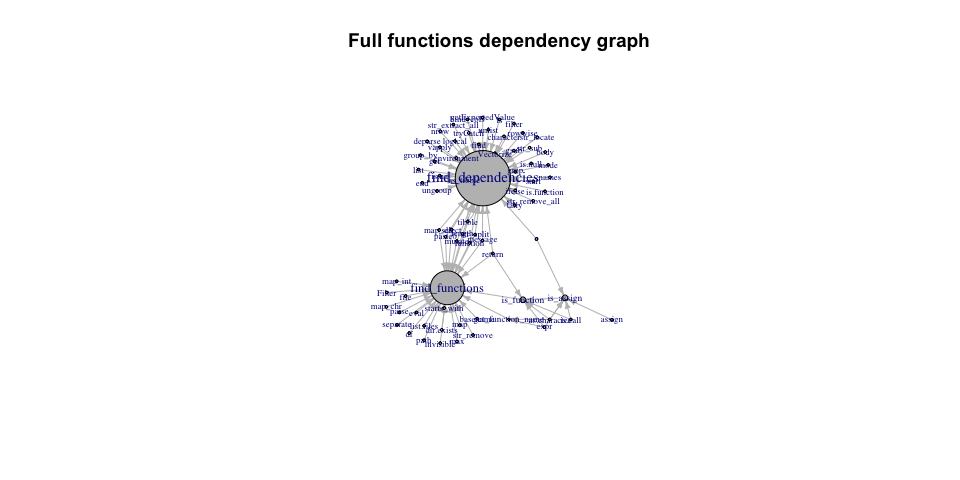# Functiondepends - usage

library(functiondepends)
# Create environment for loaded functions
envir <- new.env()
# Search recursively source files
functions <- find_functions(".", envir = envir, recursive = TRUE)
functions
#> # A tibble: 5 × 3
#>   Path  Function          SourceFile
#>   <chr> <chr>             <chr>
#> 1 R     find_dependencies find-dependencies.R
#> 2 R     is_function       find-functions.R
#> 3 R     get_function_name find-functions.R
#> 4 R     is_assign         find-functions.R
#> 5 R     find_functions    find-functions.R

Search for dependencies of function find_functions within parsed functions:

dependency <- find_dependencies("find_functions", envir = envir, in_envir = TRUE)
dependency
#> # A tibble: 2 × 5
#>   Source            SourceRep SourceNamespace Target         TargetInDegree
#>   <chr>                 <int> <chr>           <chr>                   <int>
#> 1 get_function_name         1 user-defined    find_functions              2
#> 2 is_function               1 user-defined    find_functions              2

Note that SourceNamespace column has value user-defined as the functions are searched within source of the package.

Search for all dependencies of find_functions function:

library(ggplot2)
library(dplyr)

dependency <- find_dependencies("find_functions", envir = envir, in_envir = FALSE)
dependency %>%
slice_max(SourceRep, n = 10) %>%
mutate(Source = reorder(Source, SourceRep)) %>%
ggplot(aes(x = Source, y = SourceRep, fill = SourceNamespace)) +
geom_col() +
coord_flip() +
labs(caption = "Top 10 most repeated calls in 'find_functions'.")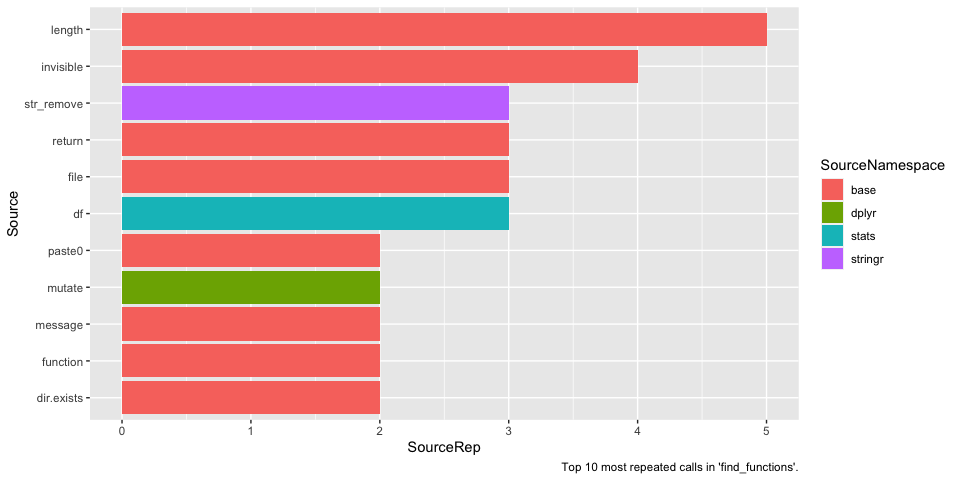Note that name df is often used to store object of type data.frame. df is also a name of F distribution density function from stats package. If you suspect that given function ought not to use a specific package, see the source code of function to check the context. To do so, one can execute find_dependencies function with add_info argument set to TRUE.

library(tidyr)

dependency <- find_dependencies("find_functions", envir = envir, in_envir = FALSE, add_info = TRUE)
dependency %>%
filter(SourceNamespace == "stats") %>%
select(Source, SourcePosition, SourceContext) %>%
unnest(c(SourcePosition, SourceContext))
#> # A tibble: 6 × 3
#>   Source SourcePosition SourceContext
#>   <chr>           <dbl> <chr>
#> 1 df                 10 "    df <- purrr::map_dfr(sourceFiles, function(file) {"
#> 2 df                 19 "    source_name <- basename(df$Path)" #> 3 df 21 " df <- df %>% dplyr::mutate(Path = stringr::str_rem… #> 4 df 23 " paths <- stringr::str_split(df$Path, \"/|\\\\\…
#> 5 df                 25 "        df <- tidyr::separate(df, \"Path\", into = pas…
#> 6 df                 27 "    df %>% dplyr::mutate(SourceFile = source_name)"

One can see that indeed df is not a call to function stats::df.

dependency <- find_dependencies(unique(functions$Function), envir = envir, in_envir = FALSE) dependency %>% distinct(Target, TargetInDegree) %>% mutate(Target = reorder(Target, TargetInDegree)) %>% ggplot(aes(x = Target, y = TargetInDegree)) + geom_col() + coord_flip() + labs(caption = "Functions with most function calls.")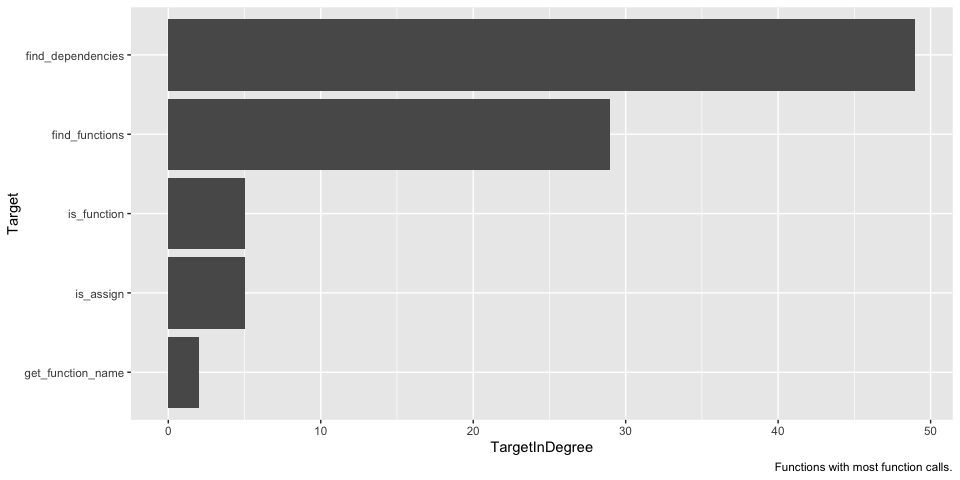dependency <- find_dependencies(unique(functions$Function), envir = envir, in_envir = FALSE)
dependency %>%
group_by(SourceNamespace) %>%
tally(name = "Count") %>%
slice_max(Count, n = 10) %>%
mutate(SourceNamespace = reorder(SourceNamespace, Count)) %>%
ggplot(aes(x = SourceNamespace, y = Count)) +
geom_col() +
coord_flip() +
labs(caption = "Top 10 used namespaces.")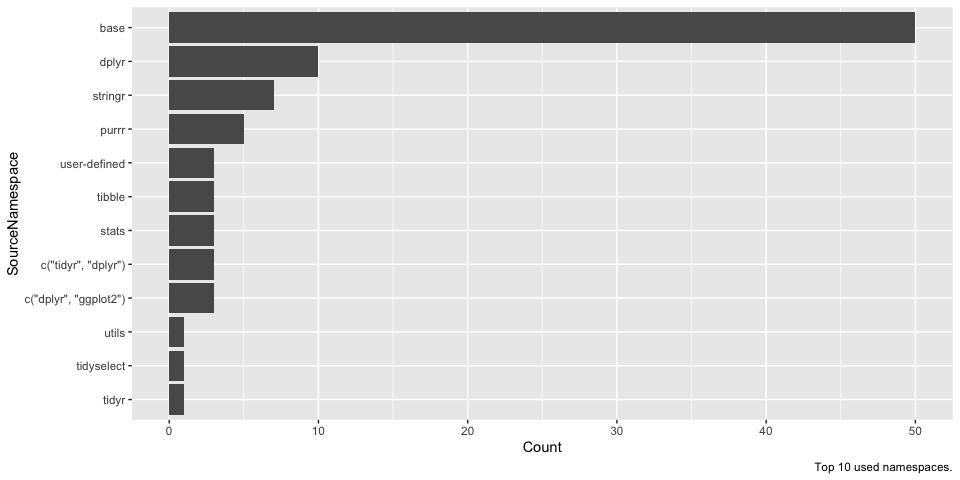See which user-defined functions depend most on other user-defined functions within searched codebase.

dependency <- find_dependencies(unique(functions$Function), envir = envir, in_envir = TRUE) dependency %>% distinct(Target, TargetInDegree) %>% arrange(-TargetInDegree) #> # A tibble: 5 × 2 #> Target TargetInDegree #> <chr> <dbl> #> 1 find_functions 2 #> 2 is_function 1 #> 3 find_dependencies 0 #> 4 get_function_name 0 #> 5 is_assign 0 library(igraph) edges <- dependency %>% select(Source, Target) %>% na.omit() vertices <- unique(c(dependency$Source, dependency$Target)) vertices <- vertices[!is.na(vertices)] g <- graph_from_data_frame(d = edges, vertices = vertices) deg <- degree(g, mode = "in") V(g)$size <- deg * 10 + 5
V(g)$label.cex <- (degree(g, mode = "in", normalized = TRUE) + 1) plot( g, vertex.color = "grey", edge.color = "grey", edge.arrow.size = .4, main = "Functions dependency graph" )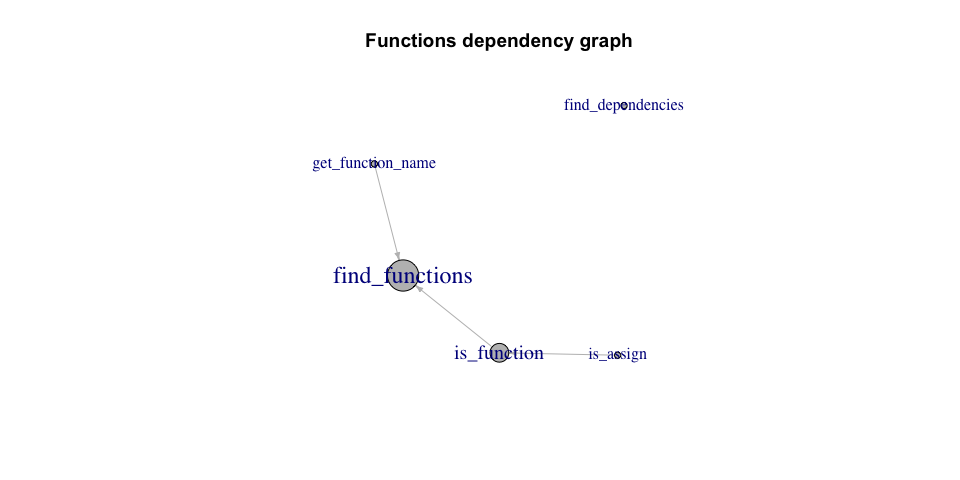dependency <- find_dependencies(unique(functions$Function), envir = envir, in_envir = FALSE)
edges <- dependency %>%
select(Source, Target) %>%
na.omit()

vertices <- unique(c(edges$Source, edges$Target))

g <- graph_from_data_frame(edges)
deg <- degree(g, mode = "in")
V(g)$size <- deg V(g)$label.cex <- (degree(g, mode = "in", normalized = TRUE) + 1) / 1.8

plot(
g,
vertex.color = "grey",
edge.color = "grey",
edge.arrow.size = .4,
main = "Full functions dependency graph"
)